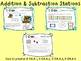# April Math StationsSubject
Resource Type
File Type
PDF (14 MB|125 pages)
Standards
\$8.25
• Product Description
• Standards
Includes 11 stations covering a wide range of kindergarten math skills with an April theme.
Counting Station: Line ‘Em Up (CC.A.1, CC.A.3)
In this activity students can line up sets of cards in order. Three sets are included (1-20, by 5s to 100, by 10s to 100)
Counting Station: Number Puzzles (CC.A.1, CC.A2)
In this activity students put together multiple puzzles in a variety of counting sequences.
Counting Station: Spring Into Math (CC.A.3, CC.B.5)
In this activity, students choose a graphing board. They have to count the number of objects on the board and then graph them on a recording sheet. There are 3 different boards to choose from.
Teen Number Puzzles (K.NBT.A.1)
In this activity, students put together puzzles that show a teen number, a base 10 representation and the teen number written in words.
Measurement Station (K.MD.A.1)
In this activity, students use a jelly bean “ruler” to measure the height and length of different pictures.
In this activity, students match equation cards with cards that show a picture to represent the subtraction facts.
Addition Station: Number Line Match (OA.A.1)
In this activity, students match equation cards with cards that show a picture to represent the addition pictures and addition number lines
In this activity, students play an adding version of the game BUMP!
Addition Station: Grow and Shrink (K.OA.A.1)
In this activity, students roll a die and place counters on a ten frame board. Students roll again and have to add to or take away from the original set.
In this game, students add the two numbers on dice to clear the board of their opponent’s counters.
Addition/Subtraction Station: Fact Family Puzzles (OA.A.1, OA.A.5)
In this activity, students match puzzles that show the 4 equations of fact families through 10.

How to get TPT credit to use on future purchases:
• Please go to your My Purchases page (you may need to login). Beside each purchase you'll see a Provide Feedback button. Simply click it and you will be taken to a page where you can give a quick rating and leave a short comment for the product. I value your feedback greatly as it helps me determine which products are most valuable for your classroom so I can create more for you. ☺

Be the first to know about my new discounts, freebies and product launches:
• Look for the green star near the top of any page within my store and click it to become a follower. You will then receive customized email updates about this store.
Fluently add and subtract within 5.
Decompose numbers less than or equal to 10 into pairs in more than one way, e.g., by using objects or drawings, and record each decomposition by a drawing or equation (e.g., 5 = 2 + 3 and 5 = 4 + 1).
Represent addition and subtraction with objects, fingers, mental images, drawings, sounds (e.g., claps), acting out situations, verbal explanations, expressions, or equations.
Describe measurable attributes of objects, such as length or weight. Describe several measurable attributes of a single object.
Compare two numbers between 1 and 10 presented as written numerals.
Total Pages
125 pages